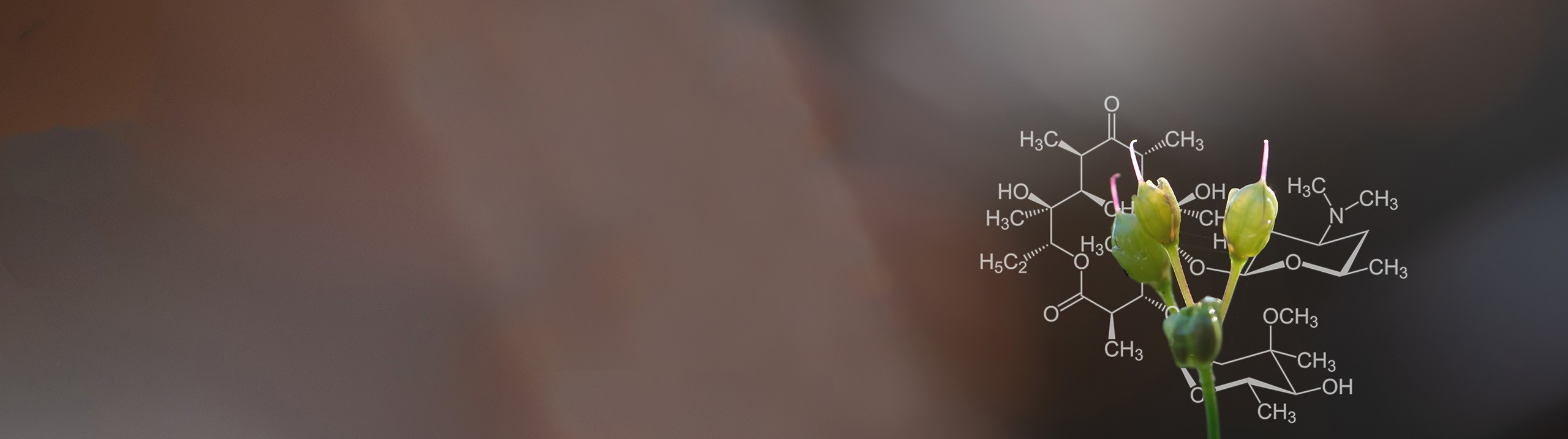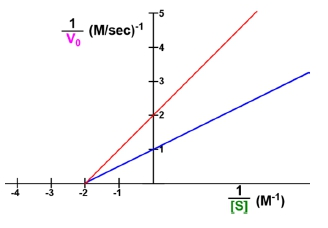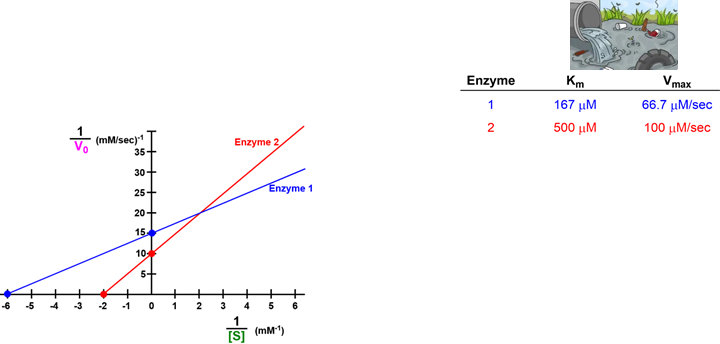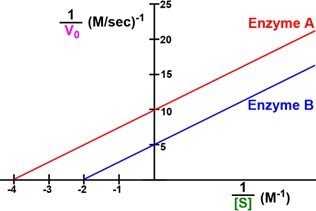Start typing, then use the up and down arrows to select an option from the list.# Biochemistry

Learn the toughest concepts covered in Biochemistry with step-by-step video tutorials and practice problems by world-class tutors

6. Enzymes and Enzyme Kinetics

# Shifting Lineweaver-Burk Plots

1

#### concept

Shifting Lineweaver-Burk Plots7m
Play a video:
in this video, we're going to talk even Mawr details about a line Weaver Burke plot specifically how to shift the line on a line Weaver Burke plot. So recall from our previous lesson videos that the line Weaver Burke equation resembles the equation of a line. And so what this means is that we could take the equation of a line, which is why equals M X plus B and substitute in enzyme kinetics variables to get the line Weaver Burke equation. And so when it comes down to it, what actually defines a line are two different factors. The first factor that defines a line is the slope of the line, or the M variable in the equation of line or the cam over the V Max ratio and the line Weaver Burke equation. And the second factor that defines a line is actually the Y intercept of the line, which is the variable B in the equation of the line and the reciprocal of the V Max and the line Weaver Burke equation. And so, if we start off with just talking about the slope of the line, recall from your previous courses that when the slope of the line is equal to zero. That means that the line is going to be completely horizontal. It's gonna be a completely horizontal line when the slope is equal to zero. And as we begin thio increase the slope as we begin to increase the value of the slope that will make the line become a steeper line, so that will make the line steeper. And so, if we take a look at this line Weaver Burke plot that we have down below notice that this horizontal red line that we see here, a sui set up above horizontal lines, will have a slope or, uh, that is going to be equal to zero. So we know that the slope of this red line or the N value is going to be equal to zero and also as we set up above, if we increase the value of the slope, that's going to make the lines steeper. So notice that this green line right here is much steeper, and so that means that it's slope must be greater than the value zero. And so, essentially, what you'll notice is that the slope is also going to be equal to the rise over the run. And so if we wanted to determine the slope of this green line right here, all we would need to do is start at any point on the line and figure out how much do we need to rise, or how much do we need to go up? And how much do we need to go? Uh, to the right, Or how much do we need to run horizontally in order? Thio, get back to a point on our line. And so if we start at this point, we could see that we need to go up one unit and to the right one unit in order to get to a point on our line, which means that the rise is going to be one and the run will also be one. And of course, 1/1 is just one. And so that means that the slope of this line is just going to be one. And so what we can see is that a line that has a slope of zero is gonna be horizontal. And if we increase the value of the slope, then we're just going to get ourselves a steeper line, as we see here now, if we were to increase the value of the slope Thio to, then here we would have a steeper line. And if we were to increase the value of the slope to three, then we would have an even steeper line so you can see the effect that increasing the slope will have on the steepness of the line. Now moving on to the Y intercept, uh, the Y intercept be increasing the value of the Y intercept is actually going to elevate. It's going to elevate the position that the line intersects the Y axis. So remember that the Y intercept is going to be the value of why when, uh, it crosses the Y intercept the Y axis, which is gonna be this horizontal axis. So now notice. Looking at this line, Weaver Burke plot. Here in the middle, we have a horizontal line, so we already know that horizontal lines are gonna have a slope equal to zero so we can go ahead and say that the slope is equal to zero. Here, however, uh noticed that this horizontal line is crossing the Y axis at a different position. Then the horizontal line that we had over here. Notice that the horizontal line on the left is crossing the Y axis specifically at the value of one. However, over here on the right, this horizontal line is crossing the Y axis at a different position at a value of two. And so what this shows is that, uh, if we increase the value of the Y intercept be, that's going to elevate the position of where the line is going to intersect the Y axis. And so, essentially, when it comes to shifting the line on a line we Verburg plot, all we need to consider is the slope of the line and the Y intercept of line. Now, the last point that I want to make that's important about shifting the lines of a line Weaver bird plot. Is that the slope of a line on a line Weaver Burke plot? Which is, of course, going to be, uh, the M, which is going to be the ratio of the cam over the V Max eso. We can say that the slope, which is K M over V max, cannot actually be zero or have a negative value, so essentially What this means is that for line Weaver Burke plots were never going to see a line Weaver Burke plot where we have a slope of zero. So we're never going to see horizontal lines like this, and we're also never going to see a, uh, a slope that has a negative value. So we're never going to see lines that go like this in this direction and decrease from the left to the right. So what we can say is that the slope, which is the camp of the V Max, is never going to be negative, and it's never going to be zero. It's always gonna have some kind of value. And so, essentially, we will always expect the lines of our line. We were Burke plot toe, have a positive value and have a positive slope, and so that makes it a little bit easier to interpret as well. And it takes away, ah, lot less factors that we need toe worry about. And so this concludes our lesson on shifting line. Weaver Burke plots by using the slope of the line and the Y intercept of a line. And in our next lesson video, we're going to talk about how to quickly look and visualize a line. Weaver Burke plot and analyzed the K M and the V Max, so I'll see you guys in that lesson video.
2

#### concept

Shifting Lineweaver-Burk Plots7m
Play a video:
and this video, we're going to talk about how to glance at lines on a line. Weaver Burke plot and quickly visualized whether there have been increases or decreases to the K M and or the V Max. And so this will be a skill that will be especially useful to you guys once we start talking about enzyme inhibitors later in our course. Now, I also want to point out that in this video you might get a little bit confused because we're going to talk about some counterintuitive stuff. But if you do get confused, hang on tight, because in our next example video, I'm gonna show you guys an example of how to apply this counterintuitive stuff. So hopefully things will start clicking. And it will make a lot more sense to you guys after our next example video. And so let's go on and get started here. So, first I want you guys to recall from our previous lesson videos that ah line Weaver Burke plot is also known as a double reciprocal plot because it plots reciprocal on the X and the Y axis on the X axis. It plots the reciprocal of the substrate concentration. And on the y axis it plots the reciprocal of the initial reaction velocity. Or if you're not. And so because both the X and Y axes and ah line Weaver Burke plot are actually reciprocal, essentially one over the substrate concentration and one over the initial reaction velocity be not. Then we can say that the substrate concentration and the initial reaction velocity themselves will actually increase towards zero. That's right, I said increase not decrease towards zero. And that is a little bit counterintuitive. So let's take a look at our image down below to begin clearing up some of this counterintuitive stuff. And so in this line, we were Burke plot down below. We're first going to focus on Lee on the X axis, and we're gonna kinda ignore the Y axis for a little while. So notice that the X axis plots the reciprocal of the substrate concentration and normally, on either side of this zero on the X axis, we expect the magnitude on the right side of the X axis to increase towards the right in that direction and on the left side of the zero on the X axis, we expect the magnitude to increase towards the left and really with a line with Herbert plot. It's the same exact deal. Except we need to remember that the line with Herbert plot is again plotting the reciprocal of the substrate concentration. And so when we're considering the entire reciprocal as a whole, it's true that the magnitude of the reciprocal will increase towards the right of the zero and it will increase towards the left of the zero. However, if we're not considering the entire reciprocal as a whole and instead we're only considering part of the reciprocal or just the substrate concentration, then we can say that the substrate concentration itself will increase in the opposite direction towards the zero marker. And so instead of increasing outwards from the zero in opposite directions, it's actually going to increase towards the zero. And so that's exactly what these arrows here are showing us that the substrate concentration itself. When we're on Lee Considering the substrate concentration, it will actually increase towards this zero marker and the same thing over here on the right on the left hand side, it will increase so substrate concentration increasing towards the zero marker. And so the zero marker here is really acting as the infinity marker for the substrate concentration itself. And that's why we have that The substrate concentration is equal to infinity at this zero marker here. And so also, what's associated with the X axis is, of course, the X intercept, which we know from our previous lesson videos, is the negative reciprocal of the K f. And so just just like the substrate concentration increases towards zero, we can also say that the K M is also going to increase towards zero. And so also on this side, the cam would also increase towards zero as well. And so recall that lines on a line with Robert Plant are always gonna have a positive slope like this. So we're never gonna have a negative slope where the X intercept actually has a positive value. And so what that means is really we don't need to even think about the K M ever being on this side of the zero. So the K M is never gonna land on this side of the zero. It's always gonna land over here, So really, we don't need to consider the K M over here and really. We don't even need to consider this substrate concentration line over here, because again, we're never gonna have lines that land theme X intercept on this side of the zero on the X axis. And so now moving on to the next axes the Y axis here that goes vertically, uh, noticed that the y axis also plots the reciprocal. And so it's true that when we're considering the entire reciprocal as a whole that it will increase going upwards. However, if we're not considering the entire reciprocal as a whole and we're on Lee considering the initial reaction velocity, it's actually going to increase in the opposite direction towards the zero mark again. And so that's why we have indicated down below that the initial reaction velocity is increasing downwards in this fashion towards the zero mark. And so the zero mark also acts as the infinity mark for the initial reaction velocity itself on a line with Herbert plot. And so, uh, this also applies. Recall that the, uh why access here is associated with the Y intercept, which is the reciprocal of the V max, which means that the V max itself in the bottom is also going to increase towards zero just like the K M on the X axis increases towards zero as well. And so essentially, what I want you guys to note here is that the why intercept of our line, which is be in the equation of the line and one over V Max in our line Weaver Burke equation will occur graphically at infinite substrate concentrations. And here we have the Infinity marker. And so really, what we can see here is that the line Weaver Burke plot or the double reciprocal plot really just allows us to visualize a law that we already knew from our previous lesson videos. And that is that the initial reaction velocity will approach the V Max when the substrate concentration approaches infinite substrate concentrations. And so, by remembering that this zero mark here acts as an infinity marker for the substrate concentration for the initial reaction velocity and for the V Max and the K M, then hopefully moving forward, that will help you guys too quickly. Visualize increases and or decreases to the K M and fee Max. And so again, if you're a little bit confused on these difficult and counterintuitive concepts hang on tight, because in our next example video, I'll show you guys how to apply thes counterintuitive concepts, so I'll see you guys there.
3

#### example

Shifting Lineweaver-Burk Plots Example 14m
Play a video:
All right. So now that we have an idea from our previous lesson video that both the K M and the V Max oven enzyme will actually increase towards the zero marker on a line Weaver Burke plot in this video we get to apply that counterintuitive idea in an example, which wants us to indicate which enzyme and each graph below has a greater K M and V Max. And so let's start with the line we Robert plot over here on the left first, and so notice that we actually have two lines on this line. Weaver Burke plot one for enzyme A and light blue and one for enzyme B and black. And so, starting with the K M here, we need to recall from our previous lesson videos that the K M is associated with the X intercept of the lines on a line Weaver bird plot and so recall that the X intercept is where the lines cross the X axis and so for enzyme be in black. The X intercept is right here and for enzyme A and light blue. The X intercept is right here. And so what we need to recall from Our previous lesson video is that the zero marker here acts as an infinity marker for the substrate concentration as well as for the K M. And it acts as an infinity marker for the initial reaction velocity as well as for the V Max. And so because the X intercept of enzyme A is closer to the infinity marker, that means that the K M of enzyme A is actually going to be greater than the K M of enzyme B, which is further away from the infinity marker. And so what that means is that we can say that enzyme A has a greater K M than enzyme be. Since it's closer to this infinity marker here and so down below, we can indicate that the K M of enzyme A is actually greater than the K m of enzyme be. And so now, moving on to the V. Max recall that the V Max is associate ID with the Y intercept of the line Weaver bird plot. And so the why intercept is where the lines cross with y axis. And so notice that both of these lines actually cross the Y axis at the same exact point. So they have the same value of the Y intercept, which means that both enzymes actually have the same V max. And so we can say that the V Max of enzyme A is exactly equal to the V max of enzyme be and so that actually concludes our answers for the first line. Weaver Burke plot So now let's move on to the line we were Burke plot on the right. And again we have two different lines. One for enzyme, see an orange and one for enzyme D here in purple. And so this time, looking at the K M. Recall that it's associated with the X intercept, which is where the lines cross the X axis and noticed that they both crossed the X axis here at the same exact point, which is indicating that the K M of both of these enzymes is exactly the same. So we can say that the cam of enzyme C is exactly equal to the K M of enzyme deep. And so now moving on to the V max, which is again associated with y intercept, we need to recall that the Y intercept is where the lines cross the Y axis. And so for enzyme. See, the Y intercept is up here and for enzyme d. The Y intercept is right here. And so because the why intercept for enzyme D is closer to zero, which again acts as the infinity marker, we can say that the V max for enzyme D is actually gonna be greater than the V Max for enzyme C, which is further away from the infinity marker. And so we can say that the V Max of enzyme see is actually gonna be smaller than the V max of enzyme D, which is greater and closer to this infinity marker. And so this here concludes our example on how to apply the counter intuitive, uh, plots for line Weaver Burke plots and double reciprocal plots, and we'll be able to apply thes counterintuitive ideas in our practice problems. So I'll see you guys there
4
Problem

Use the plot below. The Km of both enzymes for their substrate is:5
Problem

Suppose a company develops two enzymes that degrade highly toxic compounds to non-toxic compounds. Your task is to degrade the greatest amount of toxic compound in the shortest amount of time.

A) Which enzyme is better to use when [S] = 0.167 mM? ____________

B) Which enzyme is better to use at saturating [S]? ____________